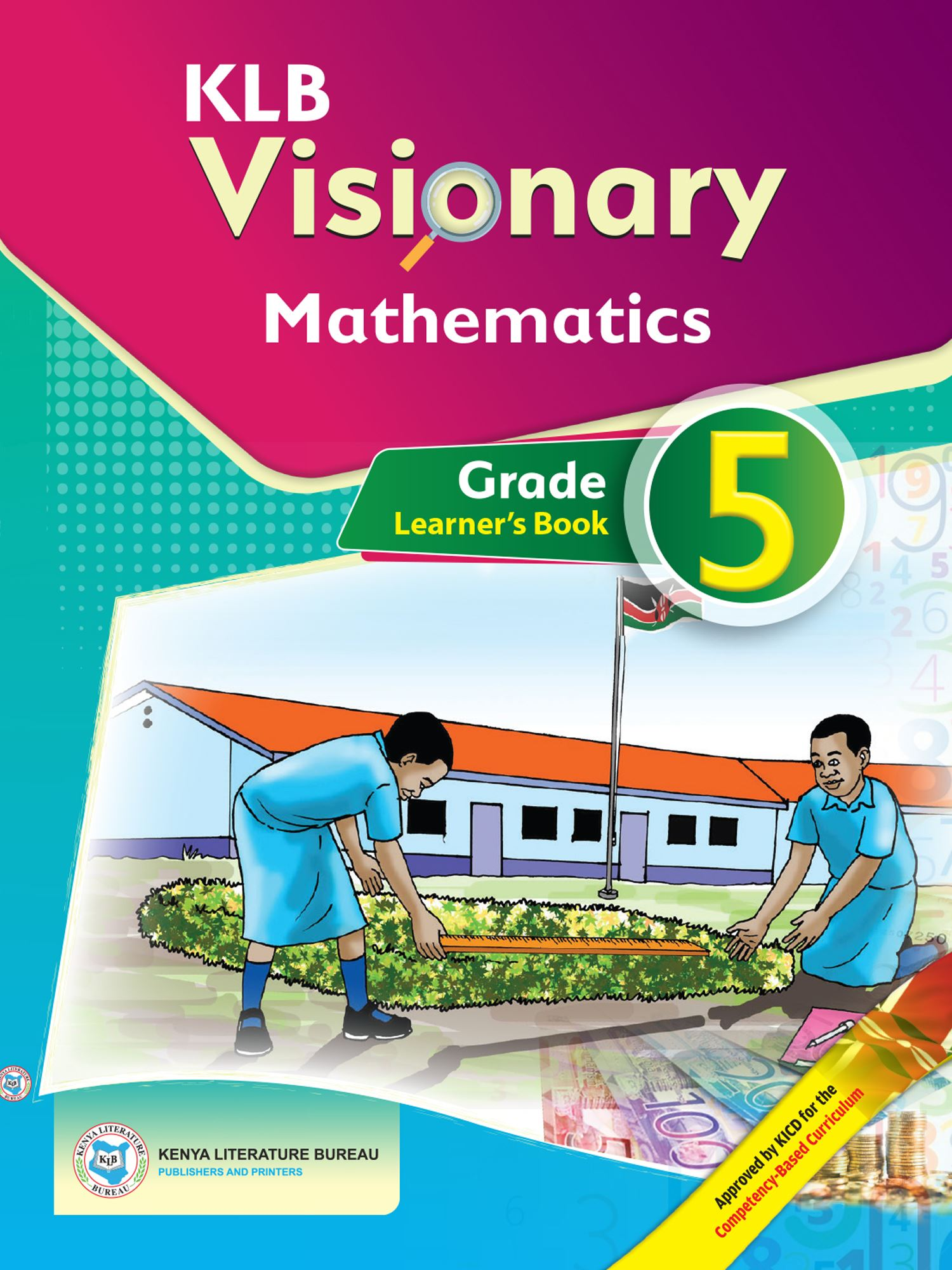Study kit (Mathematics 5)##### Authors
Obadiah Kiburi, Samuel Irumbi, Zipporah N. Muli, Francis Mwangi
##### Exercises kit’s authors
Obadiah Kiburi, Samuel Irumbi, Zipporah N. Muli, Francis Mwangi
##### Publisher
Kenya Literature Bureau
##### Included in packages• The study kit contains 100 chapters and teacher’s exercises kit contains 83 exercises.
• ##### Authors
Obadiah Kiburi, Samuel Irumbi, Zipporah N. Muli, Francis Mwangi
• ##### Exercises kit’s authors
Obadiah Kiburi, Samuel Irumbi, Zipporah N. Muli, Francis Mwangi
Mathematics
English
• ##### Publisher
Kenya Literature Bureau

### 1. Numbers – Whole Numbers

##### Chapter
1.1. Place value and total value of digits up to hundreds of thousands
Free chapter!
1.2. Reading and writing numbers up to hundreds of thousands in symbols
1.3. Reading and writing numbers in words up to tens of thousands
1.4. Ordering numbers up to tens of thousands
1.5. Rounding off numbers up to tens of thousands
1.6. Divisibility tests of 2, 5 and 10
1.7. Highest common factors (HCF) and greatest common divisors (GCD)
1.8. Least common multiples (LCM)

##### Chapter
2.1. Addition of up to three 6-digit numbers without regrouping
2.2. Addition of up to two 6-digit numbers with one regrouping
2.3. Addition of up to two 6-digit numbers with double regrouping
2.4. Estimating sums by rounding off addends
2.5. Creating number patterns involving addition

### 3. Numbers – Subtraction

##### Chapter
3.1. Subtraction of up to 6-digit numbers without regrouping
3.2. Subtraction of up to 6-digit numbers with regrouping
3.3. Estimating difference by rounding off numbers to the nearest hundreds
3.4. Estimating differences by rounding off numbers to the nearest thousand
3.5. Combined operations involving addition and subtraction
3.6. Patterns involving subtraction

### 4. Numbers – Multiplication

##### Chapter
4.1. Multiplication of a 3-digit number by a 1-digit number
4.2. Multiplication of a 3-digit number by a 2-digit number without regrouping
4.3. Multiplication of a 3-digit number by a 2-digit number with regrouping
4.4. Estimating products by rounding off factors to the nearest ten
4.5. Estimating products using friendly factors
4.6. Number patterns involving multiplication

### 5. Numbers – Division

##### Chapter
5.1. Division of a 2-digit number by a 2­digit number
5.2. Division of a 3-digit number by up to a 2-digit number
5.3. Relationship between multiplication and division
5.4. Estimating quotients by rounding off dividend and divisor to the nearest ten
5.5. Combined operations involving addition, subtraction, multiplication and division
5.6. Creating number games and puzzles involving division

### 6. Numbers – Fractions

##### Chapter
6.1. Equivalent fractions
6.2. Simplifying fractions
6.3. Comparing fractions
6.4. Ordering fractions
6.5. Addition of fractions with same denominator
6.6. Subtraction of fractions with same denominator
6.7. Addition of fractions with one renaming
6.8. Subtraction of fractions with one renaming

### 7. Numbers – Decimals

##### Chapter
7.1. Decimals up to thousandths
7.2. Place value of decimals up to thousandths
7.3. Ordering decimals up to thousandths from the smallest to the largest
7.4. Ordering decimals up to thousandths from the largest to the smallest
7.5. Addition of decimals up to thousandths
7.6. Subtraction of decimals up to thousandths

### 8. Measurement – Length

##### Chapter
8.1. Measuring distance
8.2. Metres and kilometres
8.3. Addition of metres and kilometres
8.4. Subtraction of metres and kilometres
8.5. Multiplication of metres and kilometres by whole numbers
8.6. Division of kilometres and metres by whole numbers

### 9. Measurement – Area

##### Chapter
9.1. Square centimetre
9.2. Finding area of rectangles and squares by counting one centimetre squares
9.3. Area of rectangle and square as a product of rows and columns
9.4. Area of the rectangle or square as the product of length and width

### 10. Measurement – Volume

##### Chapter
10.1. Cubic Centimetre
10.2. Volume of Cubes and Cuboids using the Formula
10.3. Volume of Cubes and Cuboids

### 11. Measurement – Capacity

##### Chapter
11.1. Millilitre
11.2. Litres and Millilitres
11.3. Conversion of Units of Capacity
11.4. Addition of Litres and Millilitres
11.5. Subtraction of Litres and Millilitres
11.6. Multiplication of Litres and Millilitres
11.7. Division of Litres and Millilitres

### 12. Measurement – Mass

##### Chapter
12.1. Measuring Mass in Grams
12.2. The Kilogram and the Gram
12.3. Addition of Grams and Kilograms
12.4. Subtraction of Grams and Kilograms
12.5. Multiplication of Grams and Kilograms
12.6. Division of Grams and Kilograms

### 13. Measurement – Time

##### Chapter
13.1. Measuring Time. Second. Minute
13.2. Conversion of units of time
13.4. Arithmetic with Time. Subtraction
13.5. Arithmetic with Time. Multiplication
13.6. Arithmetic with Time. Division

### 14. Measurement – Money

##### Chapter
14.1. Budget
14.2. Tax
14.3. Bank. Savings

### 15. Geometry – Lines – Angles

##### Chapter
15.1. Identifying and drawing horizontal and vertical lines
15.2. Perpendicular and parallel lines
15.3. Angles
15.4. Measuring angles
15.5. Measuring angles in degrees

16.1. 3D-Objects
16.2. Cuboid
16.3. Cube
16.4. Cylinder
16.5. Pyramid
16.6. Sphere

### 17. Data Handling – Data Representation

##### Chapter
17.1. Collection and Representation of Data Using Tables
17.2. Representation of Data through Piling
17.3. Interpretation of Data Represented through Piling